# Overdetermined system

A system in which the number of equations is greater than the number of unknowns. In the linear case, such a system is given by a rectangular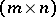-matrix,, whereis the number of equations andthe number of unknowns. The primary question for an overdetermined system is its solvability, expressed by compatibility conditions.

For example, an overdetermined system of linear algebraic equations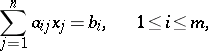is solvable if and only if the ranks of the basic matrix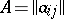and the expanded matrix obtained by adding the column of free terms tocoincide.

For an overdetermined system of linear differential equations with constant coefficients,(1)

whereis a polynomial in one variable (an ordinary differential equation) or several variables (a partial differential equation), whileis the symbol of differentiation, the compatibility condition is a homogeneous system of equations with constant coefficients:(2)

where the matrixcan be algebraically determined from the matrix.

If (1) is a system of partial differential equations with variable coefficients, it is much more difficult to determine compatibility conditions, which take the form (2) with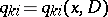.

The simplest example of an overdetermined system is the system of differential equationsThe compatibility conditions for this system, necessary and sufficient for it to be solvable, take the form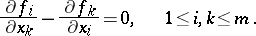An analytic function of several complex variablescan also be considered as a solution to the overdetermined system of equations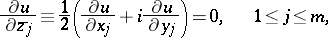where.

How to Cite This Entry:
Overdetermined system. Encyclopedia of Mathematics. URL: http://encyclopediaofmath.org/index.php?title=Overdetermined_system&oldid=33902
This article was adapted from an original article by A.P. Soldatov (originator), which appeared in Encyclopedia of Mathematics - ISBN 1402006098. See original article